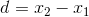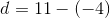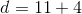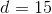# High School Math : Number Theory

## Example Questions

### Example Question #1 : Number Theory

Without using a calculator, which of the following is the best estimate for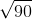?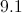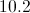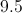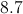Explanation:

We know that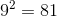and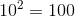.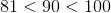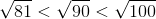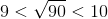Because 90 falls is approximately halfway between 81 and 100, the square root of 90 is approximately halfway between 9 and 10, or 9.5.

### Example Question #1 : Understanding Real Numbers

Place in order from smallest to largest: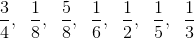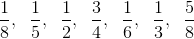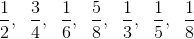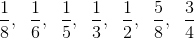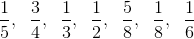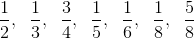Explanation:

To place in order, first we must find a common denominator and convert all fractions to that denominator.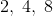have a common denominator of.have a common denominator of.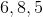have a common denominator of.

Therefore we can use common denominators to make all of the fractions look similar.  Then the ordering becomes trivial.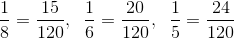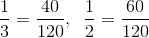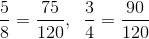### Example Question #2 : Understanding Real Numbers

What number isof?Explanation:

For percent problems there are verbal cues:

"IS" means equals and "OF" means multiplication.

Then the equation to solve becomes: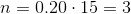### Example Question #3 : Understanding Real Numbers

Which of the following is NOT a real number?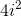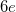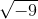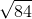Explanation:

We are looking for a number that is not real., andare irrational numbers, but they are still real.

Then,is equivalent toby the rules of complex numbers. Thus, it is also real.

That leaves us with:which in fact is imaginary (since no real number multiplied by itself yields a negative number) and simplifies to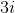### Example Question #4 : Understanding Real Numbers

Which of the following are considered real numbers?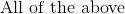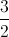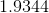Explanation:

Real numbers can be found anywhere on a continuous number line ranging from negative infinity to positive infinity; therefore, all of the numbers are real numbers.

### Example Question #1 : Understanding Number Sets

If a card is drawn randomly from a regular shuffled 52 card deck, what is the probability that the card is either a spade or a 3?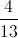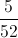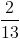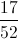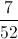Explanation:

How many cards in the deck are either a spade or a 3?

There are four 3's, including a 3 of spades.

Since we are counting the same card (3 of spades) twice, there are actually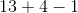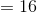distinct cards that fit the criteria of being either a spade or a 3.

Since any of the 52 cards is equally likely to be drawn, the probability that it is a spade or a 3, is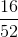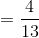### Example Question #2 : Number Lines

Find the distance betweenandon a number line.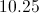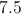Explanation:

To find the distance on a number line: